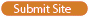# Mathematics## Home » United States » Science and Technology » Mathematics

 Site Listings previous   «     1     »   next
• CalTech - A small interactive research environment for graduate students interested in exploring the mathematical properties.
https://www.cms.caltech.edu/
• DreamBox K-3 Math Learning - Curriculum-based mathematics software for kindergarten, 1st grade, 2nd grade & 3rd grade kids.
https://www.dreambox.com/
• Math Games.org - Mathematics resource which helps children learn basic math operators through an interactive quiz.
http://mathgames.org
• National Council of Teachers of Mathematics - Association of mathematics education supporting teachers to ensure equitable mathematics learning.
https://www.nctm.org/
• NJIT - Offers a BS degree in mathematical sciences with options in applied mathematics, applied statistics, & mathematical biology.
https://math.njit.edu/
• Numerical Mathematical Utilities - Offers numerical mathematical utilities including solving N equations in N unknowns; eigenvalues and eigenvectors; and more.
http://www.akiti.ca/Mathfxns.html
• Wikipedia: Mathematics - Offers information on mathematics, how it started, and references in pure and applied mathematics.
https://en.wikipedia.org/wiki/Mathematics
 Site Listings previous   «     1     »   next